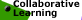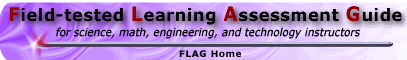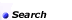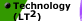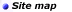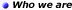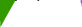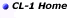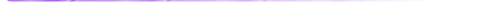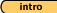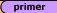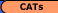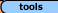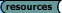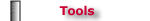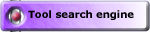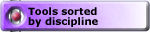## Tools - Math 'Convincing and Proving' Critiquing 'Proofs' Tasks, Set #4 (solutions)

Always, Sometimes or Never True: Set #1 (solutions) || Set #2 (solutions)
Critiquing 'Proofs': Set #3 (solutions) || Set #4 (solutions)

Malcolm Swan
Mathematics Education
University of Nottingham
Malcolm.Swan@nottingham.ac.uk

Jim Ridgway
School of Education
University of Durham
Jim.Ridgway@durham.ac.uk

The aim of this assessment is to provide the opportunity for you to:

• evaluate 'proofs' of given statements and identify which are correct;
• identify errors in 'proofs'.

1. The Pythagorean' Theorem

Here are three attempts to prove the Pythagorean theorem.

Look carefully at each attempt. Which is the best 'proof'? Explain your reasoning as fully as possible.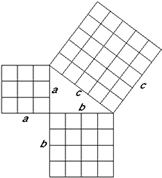Attempt 1:

Suppose a right-angled triangle has sides of length a, b and c

Draw squares on the three sides as shown.

Divide these squares into smaller squares.

You can see that the number of squares on the two shorter sides add up to make the number of squares on the longest side.

So: a2 + b2 = c2

Attempt 2:

 Suppose that you start with four right angled triangles with sides of length a, b and c and a square tray with sides of length a + b.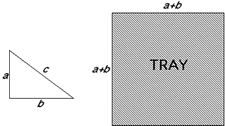You can arrange the triangles into the tray in two different ways as shown here. In the first way, you leave two square holes. These have a combined area of a2 + b2. In the second way you leave one large square hole. This has an area of c2. Since these areas are equal a2 + b2 = c2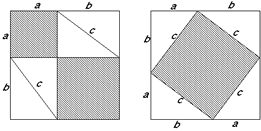Attempt 3: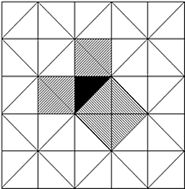The proof of the Pythagorean theorem is clear from this diagram.

The squares on the two shorter sides of the black triangle are each made from two congruent triangles.

These fit together to make the square on the longest side- the hypotenuse.

The best proof is attempt number ..........

This is because .......

My criticisms of the others are...........

Sample Solution:
Attempts 1 and 3 provide proofs for special cases only.

Attempt 1 is a sufficient demonstration where the sides of the triangle are in the ratio 3:4:5

Attempt 3 is sufficient when the sides are in the ratio 1:1: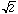Attempt 2 provides the only dissection which works for any right angled triangle.

2. Means

Here are two attempts to prove the following result about the arithmetic mean and geometric mean of two numbers:

For any positive numbers a and b,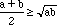Look carefully at each attempt. Which is the best 'proof'? Explain your reasoning as fully as possible.

Attempt 1:

Assuming thatMultiply both sides by 2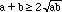Squaring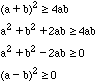Which is true for positive numbers.

So the assumption was true.

Attempt 2:

For all positive numbers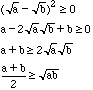So the result is true.

 Attempt 3: Look at the diagram. The area of the large square =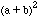(1) The unshaded area = 4ab (2) Since (1) is greater than (2)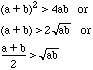So the result is true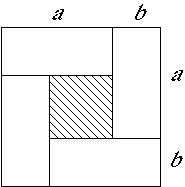The best proof is attempt number ..........

This is because .......

My criticisms of the others are...........

Sample Solution:
Attempt 1, although a logical approach, assumes what we are setting out to prove. If the argument were reversed, it would provide the basis for a proof.

Attempt 2 is correct.

Attempt 3 is the basis for a very elegant proof, but there are some holes and unnecessary jumps in it at present. The first is that it is always dangeraous to argue from adiagram because the diagram does not show all possible cases. In this example, the shaded area is clearly greater than 0 , but what if a and b were equal? In this case, the large square would be made entirely of four small squares and the shaded area would be zero. The diagram currently assumes that a > b. The special case when a = b needs to be considered. The final result thus should becomeinstead of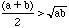. The diagram suggests that the general identity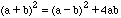would explain why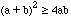for all possible values of a and b.

3. Even and Odd

Here are three attempts to prove the following result for natural numbers.

If n2 is an odd number, then n is an odd number.

Look carefully at each attempt. Which is the best 'proof'? Explain your reasoning as fully as possible.

 Attempt 1: If n was an even number, then n=2m for some m. Then n2 = 4m2 = 2(2m2) will also be an even number. So as n2 is an odd number, then n must be an odd number. Attempt 2: If n was an odd number, then since odd x odd = odd, n2 is also odd. Then n2 + n would be the sum of two odd integers and would therefore be even. Since n2 + n = n(n + 1) it is also the product of two consecutive numbers and so it certainly is even. Therefore n is odd. Attempt 3: Since n2 is odd, we have n2 = 4m2 + 4m + 1 for some m. This means n2= (2m + 1)2 for some m so n = 2m + 1 which is odd.

The best proof is attempt number ..........

This is because .......

My criticisms of the others are...........

Sample Solution:
Attempt 1 is correct.

Attempt 2 assumes what we are asked to prove (see the first line). It could, however, be modified to form a proof in the following way:

Take any positive integer n.
Let y = n(n + 1) = n2 + n.
n or n+1 must be even since they are consecutive numbers.
So y must be the product of an odd and even number. It is therefore even.
So n2 + n must be even.

Now if n2 is odd, then n (= y - n2) must also be odd. (Since even-odd = odd)

Attempt 3 does not justify why every odd square number can be expressed as 4m2 + 4m + 1 for some m.

Always, Sometimes or Never True: Set #1 (solutions) || Set #2 (solutions)
Critiquing 'Proofs': Set #3 (solutions) || Set #4 (solutions)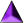Introduction || Assessment Primer || Matching Goals to CATs || CATs || Tools || Resources

Search || Who We Are || Site Map || Meet the CL-1 Team || WebMaster || Copyright || Download
College Level One (CL-1) Home || Collaborative Learning || FLAG || Learning Through Technology || NISE